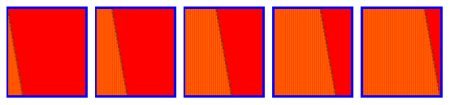#Wolfram Summer School

# Alumni# Xuxiang (Peter) Li

Summer School

Class of 2012

## Bio

Xuxiang (Peter) Li is now a graduate student at Polytechnic Institute of New York University and studied finance and risk engineering for his MSc. His favorite modules were gaming in trading and accounting, pricing derivatives, and corporate governance policies. He is interested in how prices of derivatives would evolve with stochastic variables. He is currently working as a research assistant for the journal Algorithmic Finance, for which Mr. Stephen Wolfram advises as a member of the advising board. He hopes to make a difference in education of complex financial theories through visualization using Mathematica.

## Project: SP500 Index Simulation Based on CellularAutomaton

The meaning of this project is to find a new way for simulating stock prices and/or getting its return by using cellular automata. Traditionally, this is done with three approaches:

1) Monte Carlo simulation

2) inverse function of the probability distribution of the stock prices and returns

3) a series of discrete numerical tools, such as a binomial pricing model

For the cellular automaton, Rule 30 is chosen as the generator of random numbers. Technical details are as follows:

1) CA Rule 30 functions as a random number generator.

2) The random numbers are a function of returns of the stock. The function is a polynomial of the independent variable.

3) Stock price is calculated based on each return, either by adding all the previous returns since the first time point or by multiplying all the previous (1+returns) since the first time point.

4) In this project, Rule 30 is run with different initial conditions to get a new process of stock price.

Graphs:

1) Time series plot of stock prices and returns

2) Histogram of returns and stock prices

3) Fitted distribution of the returns and stock prices

4) Comparison of results of a stochastic process and a CA generated process

## Favorite Four-Color, Four State Turing Machine

Rule 887557298582851111973699APPLY NOW